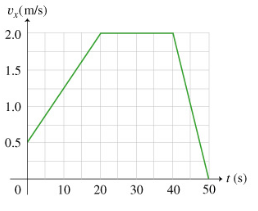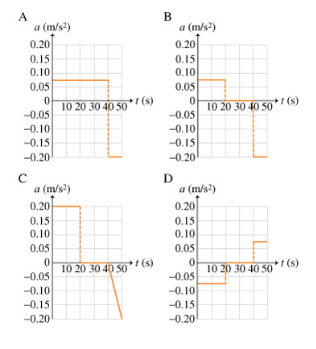# Problem: A common graphical representation of motion along a straight line is the v vs. t graph, that is, the graph of (instantaneous) velocity as a function of time. In this graph, time t is plotted on the horizontal axis and velocity v on the vertical axis. Note that by definition, velocity and acceleration are vector quantities. In straight-line motion, however, these vectors have only a single nonzero component in the direction of motion. Thus, in this problem, we will call v the velocity and a the acceleration, even though they are really the components of the velocity and acceleration vectors in the direction of motion, respectively.Part AWhat is the initial velocity of the particle, v0? Express your answer in meters per second.Part BWhat is the total distance x traveled by the particle? Express your answer in meters.Part CWhat is the average acceleration aav of the particle over the first 20.0 seconds? Express your answer in meters per second per second.Part DWhat is the instantaneous acceleration a of the particle at t=45.0s?Now that you have reviewed how to plot variables as a function of time, you can use the same technique and draw an acceleration vs time graph, that is, the graph of (instantaneous) acceleration as a function of time. As usual in these types of graphs, time t is plotted on the horizontal axis, while the vertical axis is used to indicate acceleration a.Part EWhich of the graphs shown below is the correct acceleration vs. time plot for the motion described in the previous parts?

###### FREE Expert Solution

Part A

The curve starts at 0.5 m/s

83% (287 ratings)###### Problem Details

A common graphical representation of motion along a straight line is the v vs. t graph, that is, the graph of (instantaneous) velocity as a function of time. In this graph, time t is plotted on the horizontal axis and velocity v on the vertical axis. Note that by definition, velocity and acceleration are vector quantities. In straight-line motion, however, these vectors have only a single nonzero component in the direction of motion. Thus, in this problem, we will call v the velocity and a the acceleration, even though they are really the components of the velocity and acceleration vectors in the direction of motion, respectively.Part A

What is the initial velocity of the particle, v0? Express your answer in meters per second.

Part B

What is the total distance x traveled by the particle? Express your answer in meters.

Part C

What is the average acceleration aav of the particle over the first 20.0 seconds? Express your answer in meters per second per second.

Part D

What is the instantaneous acceleration a of the particle at t=45.0s?

Now that you have reviewed how to plot variables as a function of time, you can use the same technique and draw an acceleration vs time graph, that is, the graph of (instantaneous) acceleration as a function of time. As usual in these types of graphs, time t is plotted on the horizontal axis, while the vertical axis is used to indicate acceleration a.

Part E

Which of the graphs shown below is the correct acceleration vs. time plot for the motion described in the previous parts?